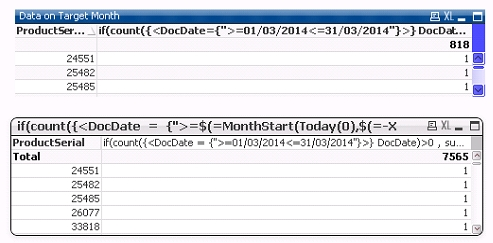QlikView App Dev

Discussion Board for collaboration related to QlikView App Development.

Announcements
QLIKWORLD LIVE! MAY 16 - 19TH, EARLY BIRD DISCOUNTS! REGISTER TODAY
cancel
Showing results for
Did you mean:Creator II

Expression Total is erratic in Pivot Table, while Straight Table is OK

Dear Gurus,

I have a strange phenomena in QV. While expression results in correct result, the total sum does not result in the correct summation per individual lines.

In expression, we have such expression.

• if(count({<DocDate={">=\$(=MonthStart(Today(0),\$(=-Y)))<=\$(=MonthEnd(Today(0),\$(=-Y)))"}>} DocDate)>0 ,sum(Weight) )

Y is an input value. Initialised as 1.

The result should be 818, but it indicates in 7,463 in Pivot Table.

If you possibly open the attached file, upper is straight table, with total mode 'Sum of Rows'. This results in 818. While Pivot Table result in 7565. In fact, if we export into Excel, we only have 818!*Since this is only on of the columns in a report in the production release, Pivot table is mandatory.

1 Solution

Accepted SolutionsEmployee

Hi Atsushi ,

This a normal behavior, in a pivot table the total is calculated always as an expression total and not as a sum of rows What you have to do to get the same result as in the straight table is to modify the expression to incorporate the sum on it like in  your modified document attached.

I hope it helps.

Carlos

2 RepliesEmployee

Hi Atsushi ,

This a normal behavior, in a pivot table the total is calculated always as an expression total and not as a sum of rows What you have to do to get the same result as in the straight table is to modify the expression to incorporate the sum on it like in  your modified document attached.

I hope it helps.

CarlosCreator II
Author

Dear Carlos,

I appreciate for the correction. Yes this is the desired result.

• sum(aggr(if(count({<DocDate = {">=\$(=MonthStart(Today(0),\$(=-X)))<=\$(=MonthEnd(Today(0),\$(=-X)))"}>} DocDate)>0 , sum(Weight) ),ProductSerial))

AtsushiCommunity Browser